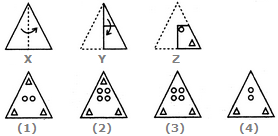# Non Verbal Reasoning - Paper Cutting - Discussion

### Discussion :: Paper Cutting - Section 1 (Q.No.7)

Each of the following questions consists of a set of three figures X, Y and Z showing a sequence of folding of apiece of paper. Figure (Z) shows the manner in which the folded paper has been cut. These three figures are followed by four answer figures from which you have to choose a figure which would most closely resemble the unfolded form of figure (Z).

7.

Choose a figure which would most closely resemble the unfolded form of Figure (Z).[A]. 1 [B]. 2 [C]. 3 [D]. 4

Explanation:

No answer description available for this question.

 Vidyansh Soni said: (Jan 7, 2018) Answer is 2.

 Biki Seth said: (Feb 11, 2018) As per my view, the answer is B.

 Lipsa Mishra said: (May 5, 2018) No the answer is C. As you observe in y, the triangular fold is gone a reside in left. If the triangle is cut at right corner it won't affect left hence the upper portion can't bear a triangle.

 Latha said: (Apr 26, 2019) I think 2 is the right one.

 Dip J Raj said: (Jun 17, 2020) 3 is right if you mind that when y is fold than the upper portion of the folding paper of triangle shape is not coincide with the lower portion, it remains only at a half portion of the last folding, so triangle not impacted on the upper portion.